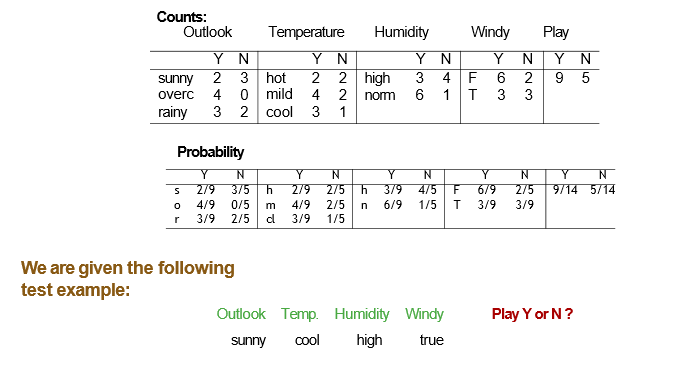top of page
Search

# Naïve Bayesian Classifiers Project & Assignment Help | What is Naive Bayesian Algorithms?

What is Naive Bayesian Algorithms?

During this lesson the following topics are covered:

• Naïve Bayesian Classifier

• Theoretical foundations of the classifier

• Use cases

• Evaluating the effectiveness of the classifier

• The Reasons to Choose (+) and Cautions (-) with the use of the classifier

Classification: assign labels to objects.

Usually supervised: training set of pre-classified examples.

Our examples:

• Naïve Bayesian

• Decision Trees

• (and Logistic Regression)

Naïve Bayesian Classifier

- Determine the most probable class label for each object

• Based on the observed object attributes

- Naïvely assumed to be conditionally independent of each other

• Example:

- Based on the objects attributes {shape, color, weight}

- A given object that is {spherical, yellow, < 60 grams}, may be classified (labeled) as a tennis ball

• Class label probabilities are determined using Bayes’ Law

- Input variables are discrete

- Output:

• Probability score – proportional to the true probability

• Class label – based on the highest probability score

Naïve Bayesian Classifier - Use Cases

- Preferred method for many text classification problems.

• Try this first; if it doesn't work, try something more complicated

- Use cases

• Spam filtering, other text classification tasks

• Fraud detection

Technical Description - Bayes' Law- C is the class label:

• C ϵ {C1, C2, … Cn}

- A is the observed object attributes

• A = (a1, a2, … am)

- P(C | A) is the probability of C given A is observed

• 4Called the conditional probability

Apply the Naïve Assumption and Remove a Constant

- For observed attributes A = (a1, a2, … am), we want to compute.and assign the classifier, Ci, with the largest P(Ci|A).

- Two simplifications to the calculations

• Apply naïve assumption - each aj is conditionally independent of each other, then• Denominator P(a1,a2,…am) is a constant and can be ignored.

Building a Naïve Bayesian Classifier

- Applying the two simplifications- To build a Naïve Bayesian Classifier, collect the following statistics from the training data:

• P(Ci) for all the class labels.

• P(aj| Ci) for all possible aj and Ci

• Assign the classifier label, Ci, that maximizes the value ofExample: Weather data setWeather data, frequency according to class:Weather example: solving our example

P(O, T , H, W | Play) = P(O | Play) · P(T | Play). P(H | Play) · P(W | Play)

Weather example when play = Yes or No:

P(Play=Y| x) = P(Play=Y) · [P(O=s| Play=Y) . P(T=c| Play=Y) . P(H=h| Play=Y) . P(W=t| Play=Y)Weather example when play = Yes:

P(Play=Y| x) = P(Play=Y) · [P(O=s| Play=Y) . P(T=c| Play=Y) . P(H=h| Play=Y) . P(W=t| Play=Y)Weather example when play = No:

P(Play=N| x) = P(Play=N) · [P(O=s| Play=N) . P(T=c| Play=N) . P(H=h| Play=N) . P(W=t| Play=N)Hire machine learning experts to get help in all machine learning related algorithms (Linear Regression, Logistic Regression, Decision Tree, K-Means, KNN, etc.).

We are providing you a 10% discount in all coding related assignments. If you are interested in giving assignments to us .We will help you out in the creativity of assignments for further details you may connect with me on WhatsApp and mail using below contact details:

----------------------------------------------------------------------------------------------------------------------